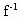我们一般用x表示自变量，用y表示函数，因此对调函数式x=(y)中字母xy．把它改写成y=(x)

定理：函数y=f(x)的图象和它的反函数y=(x)的图象关于直线y=x对称．

函数y=f(x)的定义域，是它的反函数y=(x)的值域；函数y=f(x)的值域，是它的反函数y=(x)的定义域．